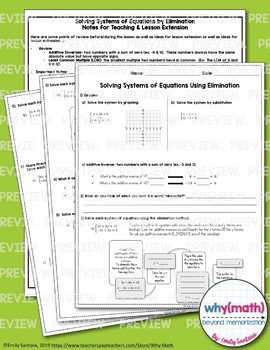# Solving Systems of Linear Equations Guided Notes, Activity, and Review BundleSubject
Resource Type
Format
Zip (68 MB|3 pages teacher notes, 18 pages student notes/activities, 16 pages answer keys)
Standards
\$8.00
Bundle
List Price:
\$10.50
You Save:
\$2.50
\$8.00
Bundle
List Price:
\$10.50
You Save:
\$2.50

### Description

Teach your students to solve systems of equations with confidence! Guided notes for four lessons, a puzzle activity, and review are included!

Guided Notes for 4 lessons for solving systems of linear equations including solving using:

• graphing
• substitution
• elimination
• elimination with multiplication (more difficult examples)

Examples of systems with one solution, no solution, and infinitely many solutions are included. Connections are made between the lessons relating the different methods of solving to one another and relating algebraic solutions to the graphs of systems. Opportunity for scaffolded student practice examples are included.

Notes for the teacher for lesson extension are included with examples of common student pitfalls with this topic. Ideas to relate new concepts with prior knowledge are also included.

Solving Systems of Equations by Graphing Puzzle Activity-

This puzzle activity allows students to practice matching a system of linear equations to the graph and the solution. This is GREAT for standardized test prep!

There are two versions to the puzzle to allow for differentiation. The first has all equations in slope-intercept form, the second has some equations which need to be converted to slope-intercept form for graphing. NOTE: Both versions have the exact same graphs and solutions, the equations are just in different forms.

One system with no solution is included in the puzzle.

Solving Systems of Equations Test (Or Review!)-

This fifteen question resource covers solving systems of equations by graphing, substitution, and elimination.

**Includes special cases of no solution and infinitely many solutions.**

This also contains three word problems that can be solved using systems of equations. Use this as a test or as a review of solving systems.

Answer Keys are included for ALL notes, both versions of the puzzle activity, and the review!

Total Pages
3 pages teacher notes, 18 pages student notes/activities, 16 pages answer keys
Included
Teaching Duration
N/A
Report this Resource to TpT
Reported resources will be reviewed by our team. Report this resource to let us know if this resource violates TpT’s content guidelines.

### Standards

to see state-specific standards (only available in the US).
Solve systems of linear equations exactly and approximately (e.g., with graphs), focusing on pairs of linear equations in two variables.
Prove that, given a system of two equations in two variables, replacing one equation by the sum of that equation and a multiple of the other produces a system with the same solutions.
Solve real-world and mathematical problems leading to two linear equations in two variables. For example, given coordinates for two pairs of points, determine whether the line through the first pair of points intersects the line through the second pair.
Solve systems of two linear equations in two variables algebraically, and estimate solutions by graphing the equations. Solve simple cases by inspection. For example, 3𝘹 + 2𝘺 = 5 and 3𝘹 + 2𝘺 = 6 have no solution because 3𝘹 + 2𝘺 cannot simultaneously be 5 and 6.
Understand that solutions to a system of two linear equations in two variables correspond to points of intersection of their graphs, because points of intersection satisfy both equations simultaneously.